# New inequalities for operator convex functions

## Abstract

The aim of this paper is to present some new inequalities of Hermite-Hadamard type inequalities for operator convex functions. In this paper, we use elementary operations and give some inequalities related to the Hermite-Hadamard type. We conclude that the results given in this work are the generalization of the recent results.

MSC:26D15, 47A63.

## 1 Introduction

Let $f:\left[a,b\right]⟶\mathbb{R}$ be a convex function, then the inequality

$f\left(\frac{a+b}{2}\right)\le \frac{1}{b-a}{\int }_{a}^{b}f\left(x\right)\phantom{\rule{0.2em}{0ex}}dx\le \frac{f\left(a\right)+f\left(b\right)}{2},\phantom{\rule{1em}{0ex}}a,b\in \mathbb{R},$
(1)

Let X be a vector space, $x,y\in X$, $x\ne y$ and $\left[x,y\right]=\left\{\left(1-t\right)x+ty,t\in \left[0,1\right]\right\}$. We consider the function $f:\left[x,y\right]⟶\mathbb{R}$ and the associated function

$g\left(x,y\right):\left[0,1\right]⟶\mathbb{R},\phantom{\rule{2em}{0ex}}g\left(x,y\right)\left(t\right):=f\left[\left(1-t\right)x+ty\right],\phantom{\rule{1em}{0ex}}t\in \left[0,1\right].$

Note that f is convex on $\left[x,y\right]$ if and only if $g\left(x,y\right)$ is convex on $\left[0,1\right]$.

For any convex function defined on a segment $\left[x,y\right]\subset X$, we have the Hermite-Hadamard integral inequality

$f\left(\frac{x+y}{2}\right)\le {\int }_{0}^{1}f\left[\left(1-t\right)x+ty\right]\phantom{\rule{0.2em}{0ex}}dt\le \frac{f\left(x\right)+f\left(y\right)}{2},$
(2)

which can be derived from the classical Hermite-Hadamard inequality (1) for the convex function $g\left(x,y\right):\left[0,1\right]⟶\mathbb{R}$.

A real-valued continuous function f on an interval I is said to be operator convex (operator concave) if

$f\left(\left(1-\lambda \right)A+\lambda B\right)\le \left(\ge \right)\phantom{\rule{0.25em}{0ex}}\left(1-\lambda \right)f\left(A\right)+\lambda f\left(B\right)$

in the operator order for all $\lambda \in \left[0,1\right]$ and for every self-adjoint operator A and B on a Hilbert space H whose spectra are contained in I. Notice that a function f is operator concave if −f is operator convex.

In recent years, many authors have been interested in giving some refinements and extensions of the Hermite-Hadamard inequality in (1). For more about convex functions and the Hermite-Hadamard inequality, see .

The author in  shows some new integral inequalities analogous to the well-known Hermite-Hadamard inequality. We give a general form of the second of these inequalities and show that the inequalities therein are satisfied for operator convex functions.

The author in  shows some new Hermite-Hadamard inequalities similar to Pachpatte’s results.

Pachpatte (2003) gives some integral inequalities analogous to the well-known Hermite-Hadamard inequality by using a fairly elementary analysis in .

Theorem 1 Let f and g be real-valued, nonnegative and convex functions on $\left[a,b\right]$. Then

1. (i)
$\frac{1}{b-a}{\int }_{a}^{b}f\left(x\right)g\left(x\right)\phantom{\rule{0.2em}{0ex}}dx\le \frac{1}{3}M\left(a,b\right)+\frac{1}{6}N\left(a,b\right),$
(3)
2. (ii)
$2f\left(\frac{a+b}{2}\right)g\left(\frac{a+b}{2}\right)\le \frac{1}{b-a}{\int }_{a}^{b}f\left(x\right)g\left(x\right)\phantom{\rule{0.2em}{0ex}}dx+\frac{1}{6}M\left(a,b\right)+\frac{1}{3}N\left(a,b\right),$
(4)

where $M\left(a,b\right)=f\left(a\right)g\left(a\right)+f\left(b\right)g\left(b\right)$, $N\left(a,b\right)=f\left(a\right)g\left(b\right)+f\left(b\right)g\left(a\right)$.

Tunç (2012) gives an inequality for convex functions in  as follows.

Theorem 2 Let $f,g:\left[a,b\right]⟶\mathbb{R}$ be two convex functions. Then(5)

where $M\left(a,b\right)=f\left(a\right)g\left(a\right)+f\left(b\right)g\left(b\right)$, $N\left(a,b\right)=f\left(a\right)g\left(b\right)+f\left(b\right)g\left(a\right)$.

Tunç (2012) gives another inequality for convex functions in , too.

Theorem 3 Let $f,g:\left[a,b\right]⟶\mathbb{R}$ be two convex functions. Then

$\begin{array}{r}\frac{1}{b-a}{\int }_{a}^{b}\left(f\left(\frac{a+b}{2}\right)g\left(x\right)+g\left(\frac{a+b}{2}\right)f\left(x\right)\right)\phantom{\rule{0.2em}{0ex}}dx\\ \phantom{\rule{1em}{0ex}}\le \frac{1}{2\left(b-a\right)}{\int }_{a}^{b}f\left(x\right)g\left(x\right)\phantom{\rule{0.2em}{0ex}}dx+\frac{1}{12}M\left(a,b\right)+\frac{1}{6}N\left(a,b\right)+f\left(\frac{a+b}{2}\right)g\left(\frac{a+b}{2}\right),\end{array}$
(6)

where $M\left(a,b\right)=f\left(a\right)g\left(a\right)+f\left(b\right)g\left(b\right)$, $N\left(a,b\right)=f\left(a\right)g\left(b\right)+f\left(b\right)g\left(a\right)$.

Ghazanfari (2012) gives an inequality for two operator convex functions in  as follows.

Theorem 4 Let $f,g:I⟶\mathbb{R}$ be operator convex functions on the interval I. Then for any self-adjoint operators A and B on a Hilbert space H with spectra in I, the inequality(7)

holds for any $x\in H$ with $\parallel x\parallel =1$, where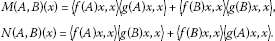For further inequalities, see .

## 2 Main results

In this section, we give some new Hermite-Hadamard type inequalities for operator convex functions and mention the differences related to the results in recent papers. We emphasize the difference by giving an example.

The following theorem is a generalization for the product of two operator convex functions.

Theorem 5 Let $f,g:I⟶\mathbb{R}$ be operator convex, nonnegative functions on the interval I. Then for any self-adjoint operators A and B with spectra in I, we have the inequality(8)

where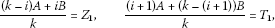(9)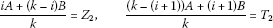(10)

and k is the number of steps.

Proof Let $x\in H$, $\parallel x\parallel =1$ and A, B be two self-adjoint operators with spectra in I. Using the convexity of f, g and the change of variable $u=kt$, we have

$\begin{array}{rl}〈f\left(\left(1-t\right)A+tB\right)x,x〉& =〈f\left(\left(1-\frac{u}{k}\right)A+\frac{u}{k}B\right)x,x〉\\ =〈f\left(\left(1-u\right)A+u\frac{\left(k-1\right)A+B}{k}\right)x,x〉\\ \le \left(1-u\right)〈f\left(A\right)x,x〉+u〈f\left(\frac{\left(k-1\right)A+B}{k}\right)x,x〉\end{array}$
(11)

and

$\begin{array}{rl}〈f\left(tA+\left(1-t\right)B\right)x,x〉& =〈f\left(\frac{u}{k}A+\left(1-\frac{u}{k}\right)B\right)x,x〉\\ =〈f\left(u\frac{A+\left(k-1\right)B}{k}+\left(1-u\right)B\right)x,x〉\\ \le u〈f\left(\frac{A+\left(k-1\right)B}{k}\right)x,x〉+\left(1-u\right)〈f\left(B\right)x,x〉.\end{array}$
(12)

By the change of variable $u=kt-1$, we have

$\begin{array}{rcl}〈f\left(\left(1-t\right)A+tB\right)x,x〉& =& 〈f\left(\left(1-\frac{u+1}{k}\right)A+\frac{u+1}{k}B\right)x,x〉\\ =& 〈f\left(\left(1-u\right)\frac{\left(k-1\right)A+B}{k}+u\frac{\left(k-2\right)A+2B}{k}\right)x,x〉\\ \le & \left(1-u\right)〈f\left(\frac{\left(k-1\right)A+B}{k}\right)x,x〉+u〈f\left(\frac{\left(k-2\right)A+2B}{k}\right)x,x〉\end{array}$

and

$\begin{array}{rcl}〈f\left(tA+\left(1-t\right)B\right)x,x〉& =& 〈f\left(\frac{u+1}{k}A+\left(1-\frac{u+1}{k}\right)B\right)x,x〉\\ =& 〈f\left(u\frac{2A+\left(k-2\right)B}{k}+\left(1-u\right)\frac{A+\left(k-1\right)B}{k}\right)x,x〉\\ \le & u〈f\left(\frac{2A+\left(k-2\right)B}{k}\right)x,x〉+\left(1-u\right)〈f\left(\frac{A+\left(k-1\right)B}{k}\right)x,x〉.\end{array}$

Similarly, by using the change of variables $u=kt-2,u=kt-3,\dots ,u=kt-\left(k-2\right)$, we have some inequalities. By the change of variable $u=kt-\left(k-1\right)$, we get

$\begin{array}{rcl}〈f\left(\left(1-t\right)A+tB\right)x,x〉& =& 〈f\left(\left(1-\frac{u+k-1}{k}\right)A+\frac{u+k-1}{k}B\right)x,x〉\\ =& 〈f\left(\left(1-u\right)\frac{A+\left(k-1\right)B}{k}+uB\right)x,x〉\\ \le & \left(1-u\right)〈f\left(\frac{A+\left(k-1\right)B}{k}\right)x,x〉+u〈f\left(B\right)x,x〉\end{array}$

and

$\begin{array}{rcl}〈f\left(tA+\left(1-t\right)B\right)x,x〉& =& 〈f\left(\frac{u+k-1}{k}A+\left(1-\frac{u+k-1}{k}\right)B\right)x,x〉\\ =& 〈f\left(uA+\left(1-u\right)\frac{\left(k-1\right)A+B}{k}x,x\right)〉\\ \le & u〈f\left(A\right)x,x〉+\left(1-u\right)〈f\left(\frac{\left(k-1\right)A+B}{k}\right)x,x〉.\end{array}$

Using the convexity of f, g, we have

$\begin{array}{rl}〈f\left(\frac{A+B}{2}\right)x,x〉& =〈f\left(\frac{tA+\left(1-t\right)B}{2}+\frac{\left(1-t\right)A+tB}{2}\right)x,x〉\\ \le \frac{〈f\left(tA+\left(1-t\right)B\right)x,x〉+〈f\left(\left(1-t\right)A+tB\right)x,x〉}{2}\end{array}$
(13)

and

$\begin{array}{rl}〈g\left(\frac{A+B}{2}\right)x,x〉& =〈g\left(\frac{tA+\left(1-t\right)B}{2}+\frac{\left(1-t\right)A+tB}{2}\right)x,x〉\\ \le \frac{〈g\left(tA+\left(1-t\right)B\right)x,x〉+〈g\left(\left(1-t\right)A+tB\right)x,x〉}{2}.\end{array}$
(14)

Firstly, if we write the values obtained from the change of variable $u=kt$ in (13) and (14), we get

$\begin{array}{rcl}〈f\left(\frac{A+B}{2}\right)x,x〉& \le & \frac{〈f\left(tA+\left(1-t\right)B\right)x,x〉+〈f\left(\left(1-t\right)A+tB\right)x,x〉}{2}\\ =& \frac{〈f\left(u\frac{A+\left(k-1\right)B}{k}+\left(1-u\right)B\right)x,x〉+〈f\left(\left(1-u\right)A+u\frac{\left(k-1\right)A+B}{k}\right)x,x〉}{2}\end{array}$
(15)

and

$\begin{array}{r}〈g\left(\frac{A+B}{2}\right)x,x〉\\ \phantom{\rule{1em}{0ex}}\le \frac{〈g\left(tA+\left(1-t\right)B\right)x,x〉+〈g\left(\left(1-t\right)A+tB\right)x,x〉}{2}\\ \phantom{\rule{1em}{0ex}}=\frac{〈g\left(u\frac{A+\left(k-1\right)B}{k}+\left(1-u\right)B\right)x,x〉+〈g\left(\left(1-u\right)A+u\frac{\left(k-1\right)A+B}{k}\right)x,x〉}{2}.\end{array}$
(16)

If we multiply (15) and (16) and suppose $\left(1-u\right)A+u\frac{\left(k-1\right)A+B}{k}={X}_{1}$ and $u\frac{A+\left(k-1\right)B}{k}+\left(1-u\right)B={Y}_{1}$, we get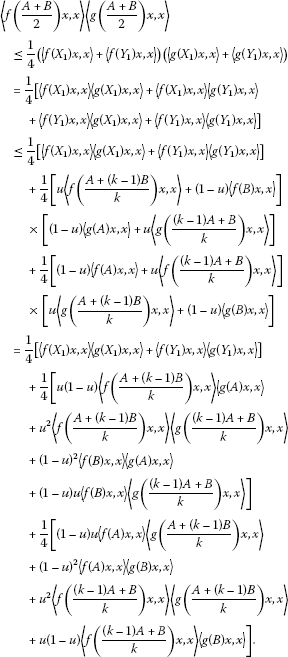(17)

If we integrate both sides of inequality (17) over $\left[0,1\right]$, we reach

$\begin{array}{r}〈f\left(\frac{A+B}{2}\right)x,x〉〈g\left(\frac{A+B}{2}\right)x,x〉\\ \phantom{\rule{1em}{0ex}}\le \frac{k}{4}\left[{\int }_{0}^{1/k}〈f\left(tA+\left(1-t\right)B\right)x,x〉〈g\left(tA+\left(1-t\right)B\right)x,x〉\phantom{\rule{0.2em}{0ex}}dt\right]\\ \phantom{\rule{2em}{0ex}}+\frac{k}{4}\left[{\int }_{0}^{1/k}〈f\left(\left(1-t\right)A+tB\right)x,x〉〈g\left(\left(1-t\right)A+tB\right)x,x〉\phantom{\rule{0.2em}{0ex}}dt\right]\\ \phantom{\rule{2em}{0ex}}+\frac{1}{24}\left[〈f\left(\frac{A+\left(k-1\right)B}{k}\right)x,x〉〈g\left(A\right)x,x〉+〈f\left(B\right)x,x〉〈g\left(\frac{\left(k-1\right)A+B}{k}\right)x,x〉\right]\\ \phantom{\rule{2em}{0ex}}+\frac{1}{12}\left[〈f\left(\frac{A+\left(k-1\right)B}{k}\right)x,x〉〈g\left(\frac{\left(k-1\right)A+B}{k}\right)x,x〉+〈f\left(B\right)x,x〉〈g\left(A\right)x,x〉\right]\\ \phantom{\rule{2em}{0ex}}+\frac{1}{24}\left[〈f\left(A\right)x,x〉〈g\left(\frac{A+\left(k-1\right)B}{k}\right)x,x〉+〈f\left(\frac{\left(k-1\right)A+B}{k}\right)x,x〉〈g\left(B\right)x,x〉\right]\\ \phantom{\rule{2em}{0ex}}+\frac{1}{12}\left[〈f\left(A\right)x,x〉〈g\left(B\right)x,x〉+〈f\left(\frac{\left(k-1\right)A+B}{k}\right)x,x〉〈g\left(\frac{A+\left(k-1\right)B}{k}\right)x,x〉\right].\end{array}$

If we continue the same operations as above until the change of variable $u=kt-\left(k-1\right)$, we have some inequalities. And then, if we sum these obtained inequalities, we get the desired inequality. □

Remark 6 In inequality (8), if we take $k=1$, we get the inequality in (7).

Now, we show the comparison between Theorems 4 and 5 utilizing self-adjoint operators (Hermitian matrices) as follows.

Example 7 Let $A=\left[\begin{array}{cc}1& 0\\ 0& 2\end{array}\right]$, $B=\left[\begin{array}{cc}-0.4& 1\\ 1& 1\end{array}\right]$. Let our operator convex functions be $f\left(X\right)={X}^{2}$ and $g\left(X\right)=X$. Since $x\in H$ and $\parallel x\parallel =1$, then we can choose x as $x=\left[\begin{array}{c}1\\ 0\end{array}\right]$. From the information given above, for $k=3$, Theorem 5 gives

$\begin{array}{r}\left({x}^{\ast }f\left(\frac{A+B}{2}\right)x\right)\left({x}^{\ast }g\left(\frac{A+B}{2}\right)x\right)\\ \phantom{\rule{1em}{0ex}}\le \frac{1}{2}{\int }_{0}^{1}〈f\left(tA+\left(1-t\right)B\right)x,x〉〈g\left(tA+\left(1-t\right)B\right)x,x〉\phantom{\rule{0.2em}{0ex}}dt\\ \phantom{\rule{2em}{0ex}}+\frac{1}{24}\left[\left({x}^{\ast }f\left(A\right)x\right)\left({x}^{\ast }g\left(\frac{A+B}{2}\right)x\right)+\left({x}^{\ast }f\left(B\right)x\right)\left({x}^{\ast }g\left(\frac{A+B}{2}\right)x\right)\\ \phantom{\rule{2em}{0ex}}+\left({x}^{\ast }f\left(\frac{A+B}{2}\right)x\right)\left({x}^{\ast }f\left(A\right)x\right)+\left({x}^{\ast }f\left(\frac{A+B}{2}\right)x\right)\left({x}^{\ast }g\left(B\right)x\right)\right]\\ \phantom{\rule{2em}{0ex}}+\frac{1}{12}\left[\left({x}^{\ast }f\left(A\right)x\right)\left({x}^{\ast }g\left(B\right)x\right)+2\left({x}^{\ast }f\left(\frac{A+B}{2}\right)x\right)\left({x}^{\ast }g\left(\frac{A+B}{2}\right)x\right)\\ \phantom{\rule{2em}{0ex}}+\left({x}^{\ast }f\left(B\right)x\right)\left({x}^{\ast }g\left(A\right)x\right)\right].\end{array}$

Putting the values of the functions in the above inequality, we get

$\begin{array}{r}0,102\le \frac{1}{2}{\int }_{0}^{1}〈f\left(tA+\left(1-t\right)B\right)x,x〉〈g\left(tA+\left(1-t\right)B\right)x,x〉\phantom{\rule{0.2em}{0ex}}dt+0.1158\\ \phantom{\rule{1em}{0ex}}⟹\phantom{\rule{1em}{0ex}}{\int }_{0}^{1}〈f\left(tA+\left(1-t\right)B\right)x,x〉〈g\left(tA+\left(1-t\right)B\right)x,x〉\phantom{\rule{0.2em}{0ex}}dt\ge -0.0276.\end{array}$

Theorem 4 gives

$\begin{array}{rcl}\left({x}^{\ast }f\left(\frac{A+B}{2}\right)x\right)\left({x}^{\ast }g\left(\frac{A+B}{2}\right)x\right)& \le & \frac{1}{2}{\int }_{0}^{1}〈f\left(tA+\left(1-t\right)B\right)x,x〉〈g\left(tA+\left(1-t\right)B\right)x,x〉\phantom{\rule{0.2em}{0ex}}dt\\ +\frac{1}{12}\left[\left({x}^{\ast }f\left(A\right)x\right)\left({x}^{\ast }g\left(A\right)x\right)+\left({x}^{\ast }f\left(B\right)x\right)\left({x}^{\ast }g\left(B\right)x\right)\right]\\ +\frac{1}{6}\left[\left({x}^{\ast }f\left(A\right)x\right)\left({x}^{\ast }g\left(B\right)x\right)+\left({x}^{\ast }f\left(B\right)x\right)\left({x}^{\ast }g\left(A\right)x\right)\right].\end{array}$

Putting the values of the functions in the above inequality, we obtain

$\begin{array}{r}0,102\le \frac{1}{2}{\int }_{0}^{1}〈f\left(tA+\left(1-t\right)B\right)x,x〉〈g\left(tA+\left(1-t\right)B\right)x,x〉\phantom{\rule{0.2em}{0ex}}dt+0.1713\\ \phantom{\rule{1em}{0ex}}⟹\phantom{\rule{1em}{0ex}}{\int }_{0}^{1}〈f\left(tA+\left(1-t\right)B\right)x,x〉〈g\left(tA+\left(1-t\right)B\right)x,x〉\phantom{\rule{0.2em}{0ex}}dt\ge -0.1386.\end{array}$

So, we can conclude that our result, Theorem 5, is more strict than Theorem 4 in this case.

The following theorem is a lower bound for the product of two operator convex functions.

Theorem 8 Let $f,g:I⟶\mathbb{R}$ be operator convex, nonnegative functions on the interval I. Then for any self-adjoint operators A and B with spectra in I, we have the inequality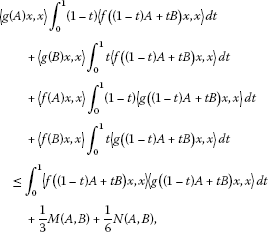(18)

where

$\begin{array}{r}M\left(A,B\right)=〈f\left(A\right)x,x〉〈g\left(A\right)x,x〉+〈f\left(B\right)x,x〉〈g\left(B\right)x,x〉,\\ N\left(A,B\right)=〈f\left(A\right)x,x〉〈g\left(B\right)x,x〉+〈f\left(B\right)x,x〉〈g\left(A\right)x,x〉.\end{array}$

Proof Let $x\in H$, $\parallel x\parallel =1$ and A, B be two self-adjoint operators with spectra in I. Define the real-valued functions ${\phi }_{x,A,B}:\left[0,1\right]⟶\mathbb{R}$ given by ${\phi }_{x,A,B}\left(t\right)=〈f\left(\left(1-t\right)A+tB\right)x,x〉$ and ${\psi }_{x,A,B}:\left[0,1\right]⟶\mathbb{R}$ given by ${\psi }_{x,A,B}\left(t\right)=〈g\left(\left(1-t\right)A+tB\right)x,x〉$. Since f and g are operator convex functions, then for every $t\in \left[0,1\right]$, we have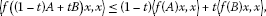(19)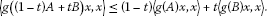(20)

If $a\le b$ and $c\le d$ for $a,b,c,d\in \mathbb{R}$, we have $ad+bc\le ac+bd$. Using this inequality analogous to (19) and (20), we get(21)

Since ${\phi }_{x,A,B}\left(t\right)$ and ${\psi }_{x,A,B}\left(t\right)$ are operator convex on $\left[0,1\right]$, they are integrable on $\left[0,1\right]$ and consequently ${\phi }_{x,A,B}\left(t\right){\psi }_{x,A,B}\left(t\right)$ is also integrable on $\left[0,1\right]$. Integrating both sides of inequality (21) over $\left[0,1\right]$, we get(22)

It can be easily controlled that

${\int }_{0}^{1}{\left(1-t\right)}^{2}\phantom{\rule{0.2em}{0ex}}dt={\int }_{0}^{1}{t}^{2}\phantom{\rule{0.2em}{0ex}}dt=\frac{1}{3},\phantom{\rule{2em}{0ex}}{\int }_{0}^{1}t\left(1-t\right)\phantom{\rule{0.2em}{0ex}}dt=\frac{1}{6}.$

When the above equalities are taken into account, the proof is complete. □

Remark 9 In inequality (18), if we take $x=\left(1-t\right)A+tB$, $a=0$ and $b=1$, we get the inequality in (5). Our result is more general than (5).

In Theorem 8, we give a lower bound. But now we give both lower and upper bounds for the product of two operator convex functions.

Theorem 10 Let $f,g:I⟶\mathbb{R}$ be operator convex, nonnegative functions on the interval I. Then for any self-adjoint operators A and B with spectra in I, we have the inequality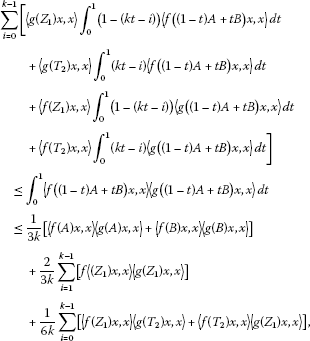(23)

where ${Z}_{1}$ and ${T}_{2}$ are defined in (9) and (10) and k is the number of steps.

Proof Let $x\in H$, $\parallel x\parallel =1$ and A, B be two self-adjoint operators with spectra in I. Using the convexity of f, g and the change of variable $u=kt$, we have (11) and (12). Using the analogous condition that, if $a\le b$ and $c\le d$ for $a,b,c,d\in \mathbb{R}$, we have $ad+bc\le ac+bd$, we obtain(24)

If we continue the same operations as above until the change of variable $u=kt-\left(k-1\right)$, we have some inequalities. And then, if we integrate the multiplication inequalities, we get k inequalities. These inequalities are defined on $\left[0,\frac{1}{k}\right),\left(\frac{1}{k},\frac{2}{k}\right),\dots ,\left(\frac{k-1}{k},1\right]$, respectively. The sum of the integration parts of these k inequalities yields ${\int }_{0}^{1}〈f\left(\left(1-t\right)A+tB\right)x,x〉〈g\left(\left(1-t\right)A+tB\right)x,x〉\phantom{\rule{0.2em}{0ex}}dt$. Thus, the proof is complete. □

Remark 11 Inequality (23) is a general form of inequality (18). When $k=1$ in inequality (23), we get inequality (18).

Theorem 12 Let $f,g:I⟶\mathbb{R}$ be operator convex, nonnegative functions on the interval I. Then for any self-adjoint operators A and B with spectra in I, we have the inequality(25)

where ${Z}_{1}$, ${Z}_{2}$, ${T}_{1}$ and ${T}_{2}$ are defined in (9) and (10) and k is the number of steps.

Proof The proof is obvious from the proofs of Theorem 3 and Theorem 5. □

Remark 13 In Theorem 12, if we take $k=1$, we get (6). Theorem 12 is a generalization of Theorem 3. If we take k as the largest number we can take in Theorem 12, we near the exact solution.

## References

1. Pecaric JE, Proschan F, Tong YL: Convex Functions, Partial Orderings and Statistical Applications. Academic Press, New York; 1991.

2. Hadamard J: Étude sur les proprietes des fonctions entieres en particulier d’une fonction considérée par Riemann. J. Math. Pures Appl. 1893, 58: 171–215.

3. Dragomir SS: Hermite-Hadamard’s type inequalities for convex functions of selfadjoint operators in Hilbert spaces. Linear Algebra Appl. 2012, 436: 1503–1515. 10.1016/j.laa.2011.08.050

4. Dragomir SS: Operator inequalities of the Jensen, Cebysev and Grüss type. Springer Briefs Math. 2012. doi:10.1007/978–1-4614–1521–3_2

5. Dragomir SS: New Hermite-Hadamard-type inequalities for convex functions (II). Comput. Math. Appl. 2011, 62: 401–418. 10.1016/j.camwa.2011.05.023

6. Dragomir SS: New refinements of the Hermite-Hadamard integral inequality for convex functions and applications. Soochow J. Math. 2002, 28(4):357–374.

7. Pachpatte, BG: On some inequalities for convex functions. RGMIA Res. Rep. Coll. 6(E) (2003)

8. Tunç M: On some new inequalities for convex functions. Turk. J. Math. 2012, 36: 245–251.

9. Ghazanfari, AG: Some new Hermite-Hadamard type inequalities for two operator convex functions (4 July 2012). http://arxiv.org/pdf/1207.0928.pdf

10. Dragomir SS: Hermite-Hadamard’s type inequalities for operator convex functions. Appl. Math. Comput. 2011, 218: 766–772. 10.1016/j.amc.2011.01.056

11. Zabandan G: A new refinement of the Hermite-Hadamard inequality for convex functions. J. Inequal. Pure Appl. Math. 2009., 10(2): Article ID 45

12. Mitrinovic DS: Analytic Inequalities. Springer, Berlin; 1970.

## Acknowledgements

This study was supported by the Coordinatorship of Selçuk University’s Scientific Research Project (BAP) and the Scientific and Technical Research Council of Turkey (TÜBİTAK). The authors would like to thank the referees for the very helpful comments and suggestions to improve this paper.

## Author information

Authors

### Corresponding author

Correspondence to Vildan Bacak.

### Competing interests

The authors declare that they have no competing interests.

### Authors’ contributions

The paper is a joint work of all the authors who contributed equally to the final version of the paper. All authors read and approved the final manuscript.

## Rights and permissions

Reprints and Permissions

Bacak, V., Türkmen, R. New inequalities for operator convex functions. J Inequal Appl 2013, 190 (2013). https://doi.org/10.1186/1029-242X-2013-190